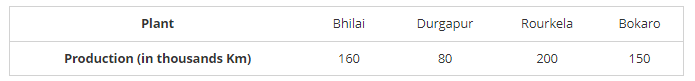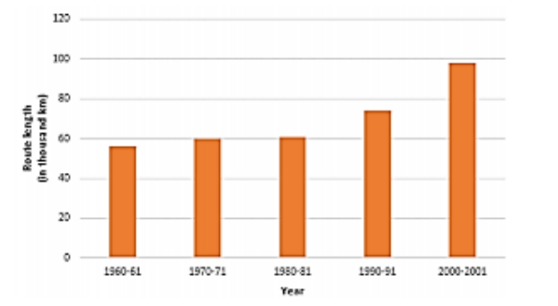# The following table gives the route length (in thousand kilometres) of the Indian Railways in some of the years:Question:

The following table gives the route length (in thousand kilometres) of the Indian Railways in some of the years:Represent the above data with the help of a bar graph.

Solution:

To represent the given data by a vertical bar graph, we first draw horizontal and vertical axes. Let us consider that the horizontal and vertical axes represent the years and the route lengths in thousand km respectively. We have to draw 5 bars of different lengths given in the table.

At first we mark 5 points in the horizontal axis at equal distances and erect rectangles of the same width at these points. The heights of the rectangles are proportional to the route lengths.

The vertical bar graph of the given data is following: